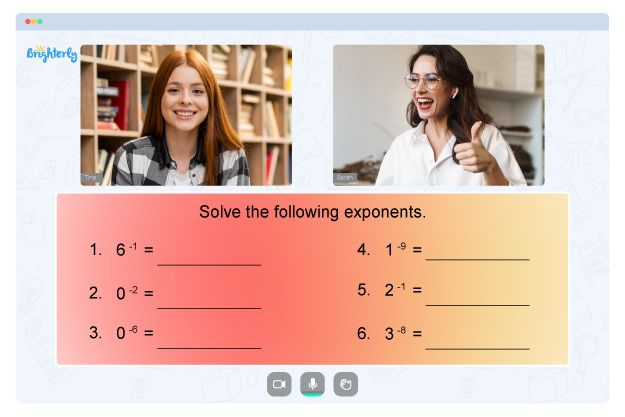# Evaluating Expressions Worksheets

In mathematics, evaluating expressions is a fundamental skill that lays the groundwork for more advanced concepts such as equations, functions, and problem-solving techniques. To develop proficiency in this area, teachers and parents often turn to evaluating algebraic expressions worksheets grade 6 as invaluable tools.

These worksheets provide structured practice opportunities, allowing learners to enhance their computational and analytical abilities. This article will explore the significance of evaluating expression worksheets, their use, and their benefits in fostering mathematical growth.

Evaluating expressions worksheets are educational resources designed to engage students in simplifying and calculating the value of mathematical expressions. The 6th grade evaluating expressions worksheets typically consist of a series of problems, each containing an expression comprising numbers, variables, and mathematical operations.

The main objective is to substitute given values into the expressions and solve them to obtain the numerical value.

## How to Use Evaluating Expressions Worksheets

### Introduction and Explanation

Before diving into evaluating algebraic expressions worksheets, provide students with a brief introduction to evaluating expressions is essential. Explain the basic components of an expression and emphasize the importance of understanding the order of operations (BODMAS) to ensure accurate calculations.

### Step-by-Step Guidance

Break down the process of free evaluating expressions worksheets into manageable steps. Start with simple examples, demonstrating how to substitute values for variables and perform necessary calculations. Then, gradually increase the complexity of expressions as students gain confidence.

### Practice Worksheets

Distribute evaluating expressions worksheets among students. Ensure the evaluating expressions worksheets PDF are age and skill-appropriate and align with the curriculum requirements. These worksheets should include various expression types, such as linear, quadratic, and exponential, and expose learners to diverse problem-solving scenarios.

### Independent Work

Allow students to work on worksheets independently and encourage them to show their work, highlighting each step to evaluate the expression. It will promote critical thinking and help identify any misconceptions or errors.

## Benefits of Evaluating Expressions Worksheets

Math for Kids

Is Your Child Struggling With Math?
1:1 Online Math Tutoring### Conceptual Understanding

Through worksheets, students gain a deeper understanding of mathematical concepts, including the relationships between variables, the significance of parentheses, and the order of operations. By exploring a range of expressions, learners can generalize their knowledge and apply it to new situations.

### Problem-Solving Skills

Evaluating expressions involves critical thinking and logical reasoning. Worksheets evaluating algebraic expressions challenge students to analyze the given expression, connect different mathematical operations, and select appropriate strategies to arrive at the correct solution. It enhances their problem-solving skills and fosters a resilient mindset.

### Individualized Learning

Evaluating numerical expressions worksheets 5th grade offer a flexible learning experience catering to the diverse needs of 5th-grade students. Teachers can tailor the difficulty level of worksheets to match individual skill levels, ensuring that each student receives appropriate challenges and support. This individualized approach fosters personalized learning and maximizes student engagement.

Problems with Numbers?• Is your child having trouble grasping numbers lessons?
• An online tutor could provide the necessary assistance.

Does your child need extra assistance with mastering numbers basics? Start learning with an online tutor.